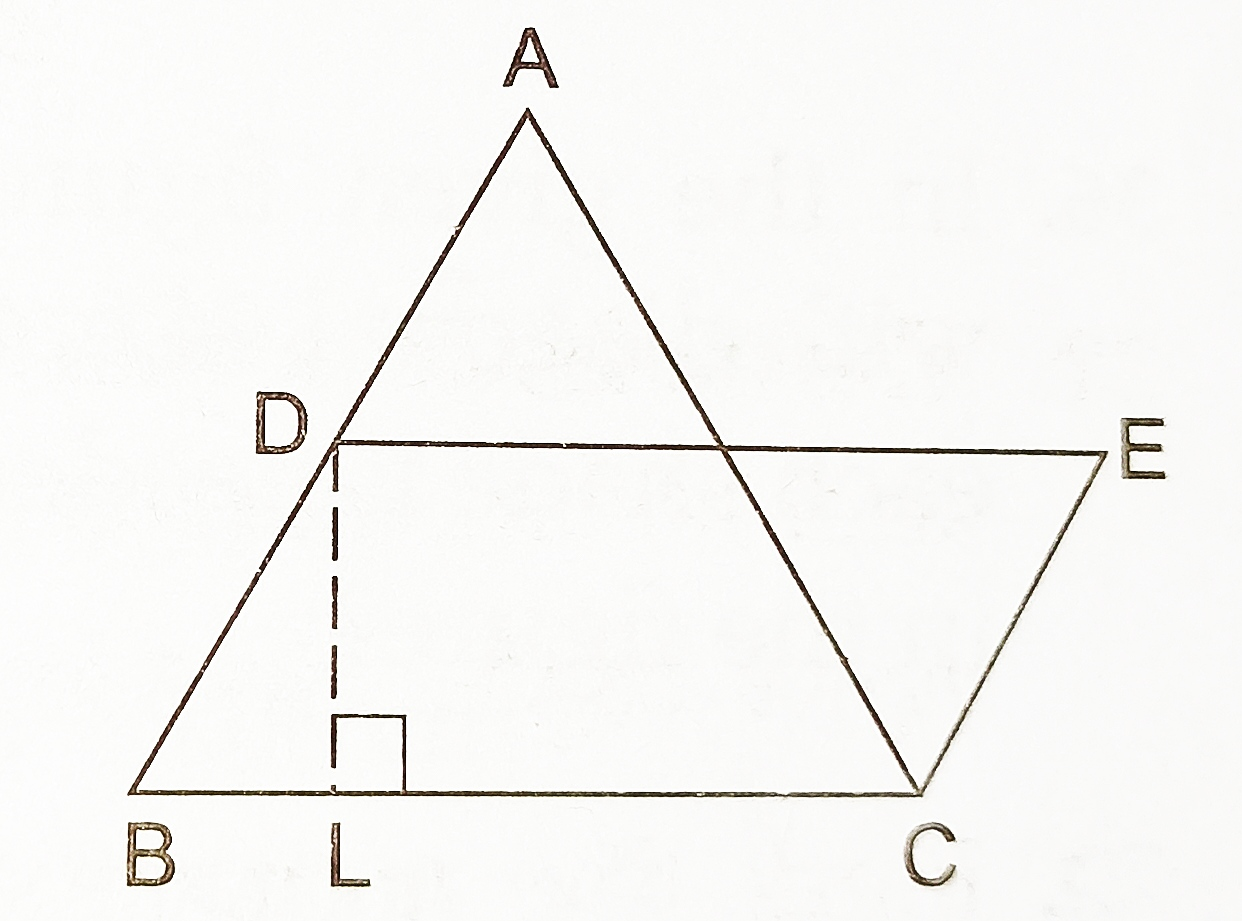# Class 9 Heron’s Formula Important Questions

Heron’s Formula – Important Questions

1. The sides of a triangular plot are in the ratio of 6 : 7 : 8 and its perimeter is 420 m. Find its area.
2. The height of an equilateral triangle is 6 cm. Find its area.
3. Find the area of a right-angled triangle whose base is 12 cm and height is 5 cm.
4. Find the area of a trapezium whose parallel sides are 11 m and 25 m long, and the nonparallel sides are 15 m and 13 m long.
5. If each side of a triangle is doubled then find the ratio of the area of the new triangle thus formed and the given triangle.
6. A triangular park ABC has sides 120 m, 80 m and 50 m (see Figure). A gardener has to put a fence all around it and also plant grass inside. How much area does she need to plant? Find the cost of fencing it with barbed wire at the rate of Rs 20 per metre leaving a space 3 m wide for a gate on one side.7. A field is in the shape of a trapezium whose parallel sides are 25 m and 10 m. The non-parallel sides are 14 m and 13 m. Find the area of the field.
8. Sides of a triangle are in the ratio of 12: 17: 25 and its perimeter is 540 cm. Find its area.
9. Find the cost of laying grass in a triangular field of sides 50 m, 65 m and 65 m at the rate of Rs 7 per m2 .
10. The length and breadth of a rectangular part are in the ratio 8:5. A path, 1.5 m wide, running all around the outside of of the park has an area of 594 m2 . Find the dimensions of the park.
11. Two sides of a triangular field are 85 m and 154 m in length and its perimeter is 324 m. Find (i) the area of the field and (ii) the length of the perpendicular from the opposite vertex on the side measuring 154 m.
12. If the area of an equilateral triangle is 363 cm2 , find its perimeter.
13. In the given figure, a ΔABC has been given in which AB = 7.5 cm, AC = 6.5 cm and BC = 7 cm. On base BC, a parallelogram DBCE of the same area as that of ABC is constructed. Find the height DL of the parallelogram.14. The lengths of the sides of a triangle are in the ratio 3 : 4 : 5 and its perimeter is 144 cm. Find (i) the area of the triangle and (ii) the height corresponding to the longest side.
15. Find the area of the parallelogram ABCD in which BC = 12 cm, CD = 17 cm and BD = 25 cm. Also, find the length of the altitude AE from vertex A on the side BC.
16. A field is in the shape of a trapezium having parallel sides 90 m and 30 m. These sides meet the third side at right angles. The length of the fourth side is 100 m. If it costs 5 to plough 1 m2 of the field, find the total cost of ploughing the field.
17. The difference between the semiperimeter and the sides of a ΔABC are 8 cm, 7 cm and 5 cm respectively. Find the area of the triangle.